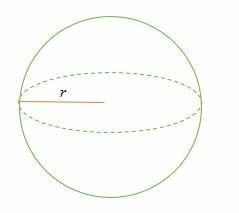# Surface Area of a Sphere – Explanation & Examples

The sphere is one of the important 3d figures in geometry. To recall, a sphere is a 3-dimensional object whereby every point is equidistance (same distance) from a fixed point, known as the sphere’s center. The diameter of a sphere divides it into two equal halves, called hemispheres.The surface area of a sphere is the measure of the region covered by the surface of a sphere.

In this article, you will learn how to find the surface area of a sphere using the surface area of a sphere formula.

## How to Find the Surface Area of a Sphere?

Like a circle, the distance from the center of a sphere to the surface is known as the radius. The surface area of a sphere is four times the area of the circle with the same radius.

### Surface area of a sphere formula

The surface area of a sphere formula is given as:

Surface area of a sphere = 4πrsquare units ……………. (Surface area of a sphere formula)

For a hemisphere (a half of a sphere), the surface area is given by;

Surface area of a hemisphere = ½ × surface area of sphere + area of the base (a circle)

= ½ × 4π r2 + π r

Surface of a hemisphere = 3πr2 …………………. (Surface area of a hemisphere formula)

Where r = the radius of the given sphere.

Let’s solve a few example problems about the surface area of a sphere.

Example 1

Calculate the surface area of a sphere of radius 14 cm.

Solution

Given:

By the formula,

Surface area of a sphere = 4πr

On substitution, we get,

SA = 4 x 3.14 x 14 x 14

= 2,461.76 cm2.

Example 2

The diameter of a baseball is 18 cm. Find the surface area of the ball.

Solution

Given,

Diameter = 18 cm ⇒ radius = 18/2 = 9 cm

A baseball has a spherical shape, therefore,

The surface area = 4πr

= 4 x 3.14 x 9 x 9

SA = 1,017.36 cm2

Example 3

The surface area of a spherical object is 379.94 m2. What is the radius of the object?

Solution

Given,

SA = 379.94 m2

But, surface area of a sphere = 4πr

⇒ 379.94 = 4 x 3.14 x r2

⇒ 379.94 =12.56r2

Divide both sides by 12.56 and then find the square of the result

⇒ 379.94/12.56 = r2

⇒ 30.25 = r2

⇒ r = √30.25

= 5.5

Therefore, the radius of the spherical solid is 5.5 m.

Example 4

The cost of leather is $10 per square meter. Find the cost of manufacturing 1000 footballs of radius 0.12 m. Solution First, find the surface area of a ball SA = 4πr = 4 x 3.14 x 0.12 x 0.12 = 0.181 m2 The cost of manufacturing a ball = 0.181 m2 x$10 per square meter

= $1.81 Therefore, the total cost of manufacturing 1000 balls =$1.81 x 1000

= $1,810 Example 5 The radius of the Earth is said to be 6,371 km. What is the surface area of the Earth? Solution The Earth is a sphere. SA = 4πr = 4 x 3.14 x 6,371 x 6,371 = 5.098 x 108 km2 Example 6 Calculate the surface area of a solid hemisphere of radius 10 cm. Solution Given: Radius, r = 10 cm For a hemisphere, the surface area is given by: SA = 3πr2 Substitute. SA = 3 x 3.14 x 10 x 10 = 942 cm2 So, the surface area of the sphere is 942 cm2. Example 7 The surface area of a solid hemispherical object is 150.86 ft2. What is the diameter of the hemisphere? Solution Given: SA = 150.86 ft2. Surface area of a sphere = 3πr2 ⇒ 150.86 = 3 x 3.14 x r2 ⇒ 150.86 = 9.42 r2 Divide both sides by 9.42 to get, ⇒ 16.014 = r2 r = √16.014 = 4 Hence, the radius is 4 ft, but the diameter is twice the radius. So, the diameter of the hemisphere is 8 ft. Example 8 Calculate the surface area of a sphere whose volume is 1,436.03 mm3. Solution Since, we already know that: Volume of a sphere = 4/3 πr3 1,436.03 = 4/3 x 3.14 x r3 1,436.03 = 4.19 r3 Divide both sides by 4.19 r3 = 343 r = 3√343 r = 7 So, the radius of the sphere is 7 mm. Now calculate the surface area of the sphere. Surface area of a sphere = 4πr = 4 x 3.14 x 7 x 7 = 615.44 mm2. Example 9 Calculate the surface area of a globe of radius 3.2 m Solution Surface area of a sphere = 4π r2 = 4π (3.2)2 = 4 × 3.14 × 3.2 × 3.2 = 128.6 m2 Hence, the surface area of the globe is 128.6 m2. ### Practice Questions 1. What is the total surface area of a sphere with a radius of$28$yards? (Use$\pi = \dfrac{22}{7}$) 2. The diameter of a spherical flask is$20$feet. What is the surface area of the flask? (Use$\pi \approx 31.4$) 3. The surface area of a spherical object is$154$ft2. What is the radius of the object? (Use$\pi = \dfrac{22}{7}$) 4. The cost of gold-plating pieces of jewelry is$\$5.60$ per square meter. How much would it cost to create $2000$ gold-plated and spherical pieces of jewelry with a radius of $0.2$ meter each? (Use $\pi \approx 3.14$)

5. The surface area of a solid hemispherical object is $1356.48$ ft2. What is the diameter of the hemisphere? (Use $\pi \approx 3.14$)